Miscellaneous

Chapter 9 Class 11 Sequences and Series (Term 1)
Serial order wise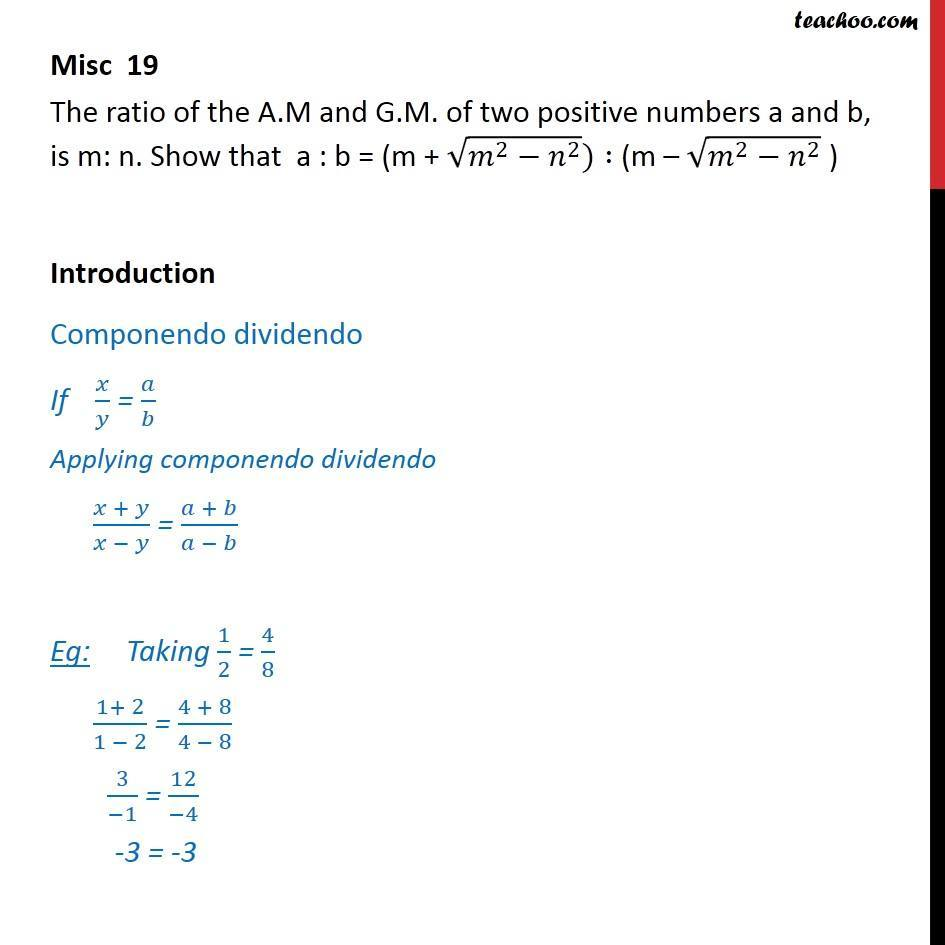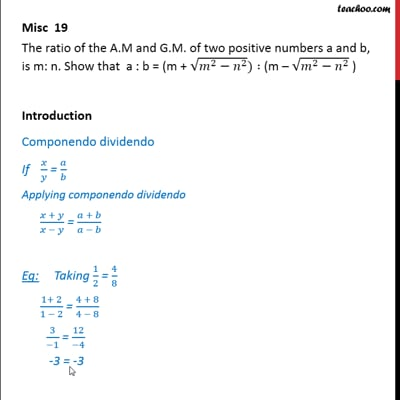This video is only available for Teachoo black users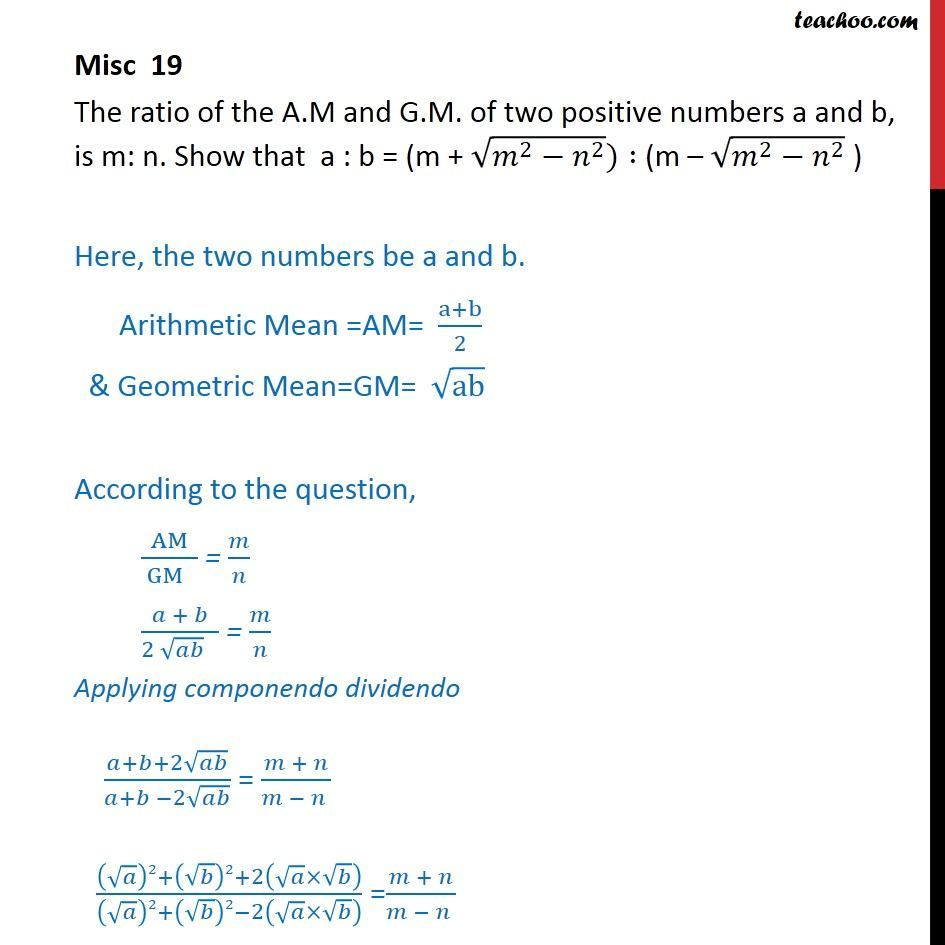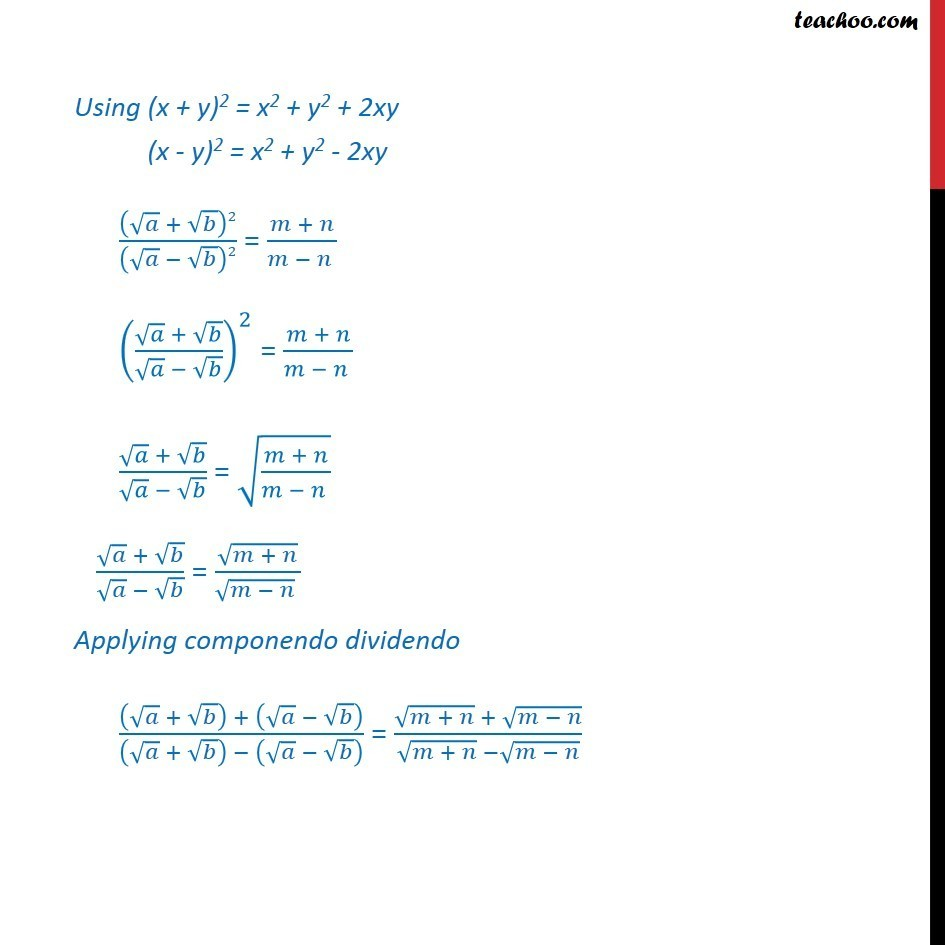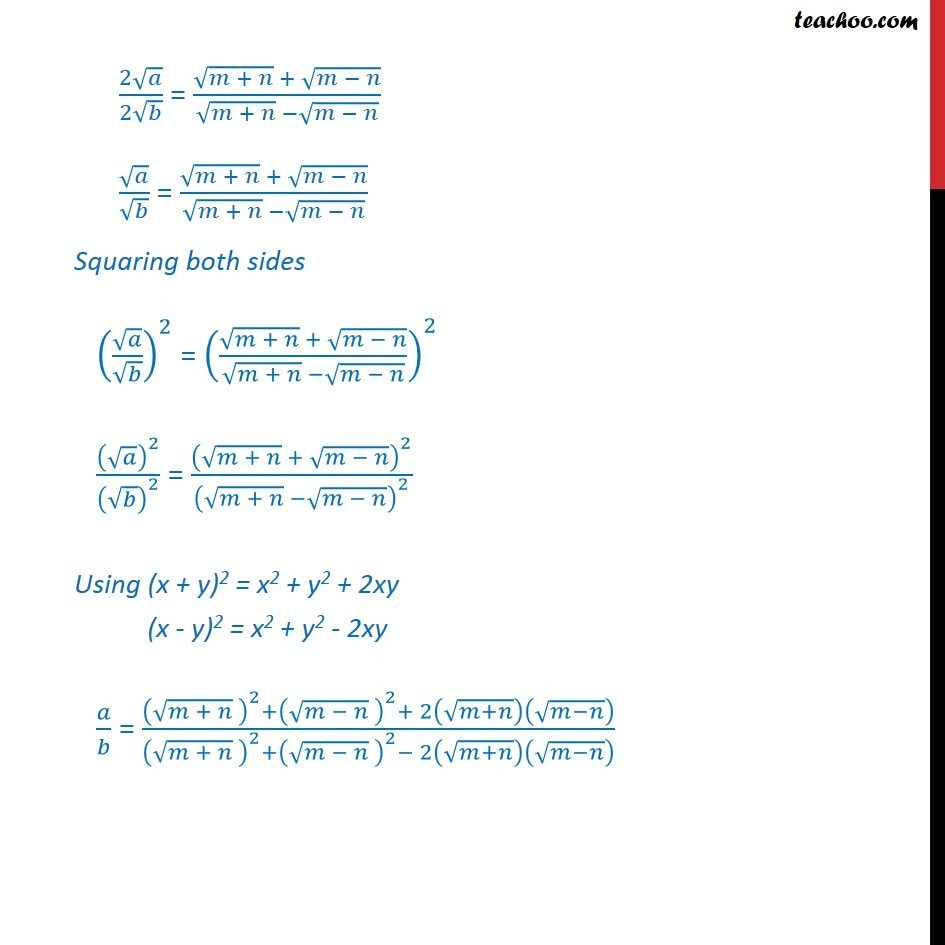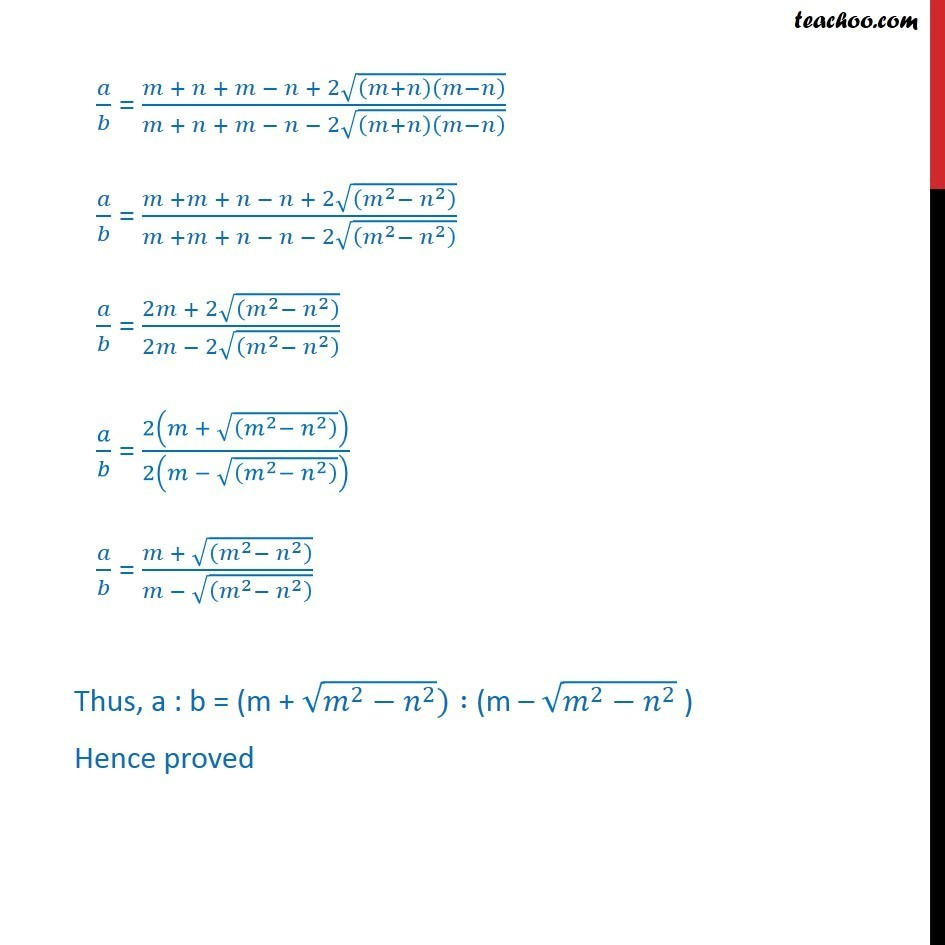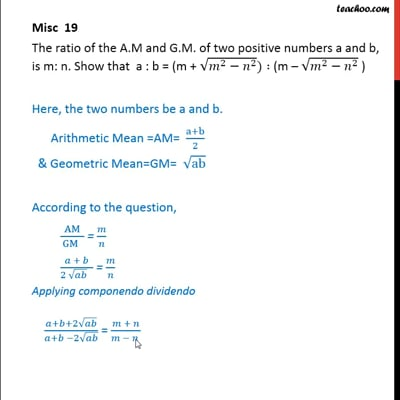This video is only available for Teachoo black users

### Transcript

Misc 19 The ratio of the A.M and G.M. of two positive numbers a and b, is m: n. Show that a : b = (m + ( ^2 ^2 )) : (m ( ^2 ^2 ) ) Introduction Componendo dividendo If / = / Applying componendo dividendo ( + )/( ) = ( + )/( ) Eg: Taking 1/2 = 4/8 (1+ 2)/(1 2) = (4 + 8)/(4 8) 3/( 1) = 12/( 4) -3 = -3 Misc 19 The ratio of the A.M and G.M. of two positive numbers a and b, is m: n. Show that a : b = (m + ( ^2 ^2 )) : (m ( ^2 ^2 ) ) Here, the two numbers be a and b. Arithmetic Mean =AM= (a+b)/2 & Geometric Mean=GM= ab According to the question, AM/( GM" " ) = / ( + )/(2 " " ) = / Applying componendo dividendo ( + +2 )/( + 2 ) = ( + )/( ) (( )2+( )2+2( ))/(( )2+( )2 2( ) ) =( + )/( ) Using (x + y)2 = x2 + y2 + 2xy (x - y)2 = x2 + y2 - 2xy ( + )2/( )2 = ( + )/( ) (( + )/( ))^2 = ( + )/( ) ( + )/( ) = (( + )/( )) ( + )/( ) = ( + )/( ( ) ) Applying componendo dividendo (( + ) + ( ))/(( + ) ( ) ) = ( ( + ) + ( ))/( ( + ) ( )) (2 )/(2 ) = ( ( + ) + ( ))/( ( + ) ( )) / = ( ( + ) + ( ))/( ( + ) ( )) Squaring both sides ( / )^2 = (( ( + ) + ( ))/( ( + ) ( )))^2 ( )^2/( )^2 = ( ( + ) + ( ))^2/( ( + ) ( ))^2 Using (x + y)2 = x2 + y2 + 2xy (x - y)2 = x2 + y2 - 2xy / = (( ( + ) )^2+( ( ) )^2+ 2( ( + ))( ( )))/(( ( + ) )^2+( ( ) )^2 2( ( + ))( ( )) ) / = ( + + + 2 (( + )( ) ))/( + + 2 (( + )( ) )) / = ( + + + 2 (( ^2 ^2 ) ))/( + + 2 (( ^2 ^2 ) )) / = (2 + 2 (( ^2 ^2 ) ))/(2 2 (( ^2 ^2 ) )) / = 2( + (( ^2 ^2 ) ))/2( (( ^2 ^2 ) )) / = ( + (( ^2 ^2 ) ))/( (( ^2 ^2 ) )) Thus, a : b = (m + ( ^2 ^2 )) : (m ( ^2 ^2 ) ) Hence proved